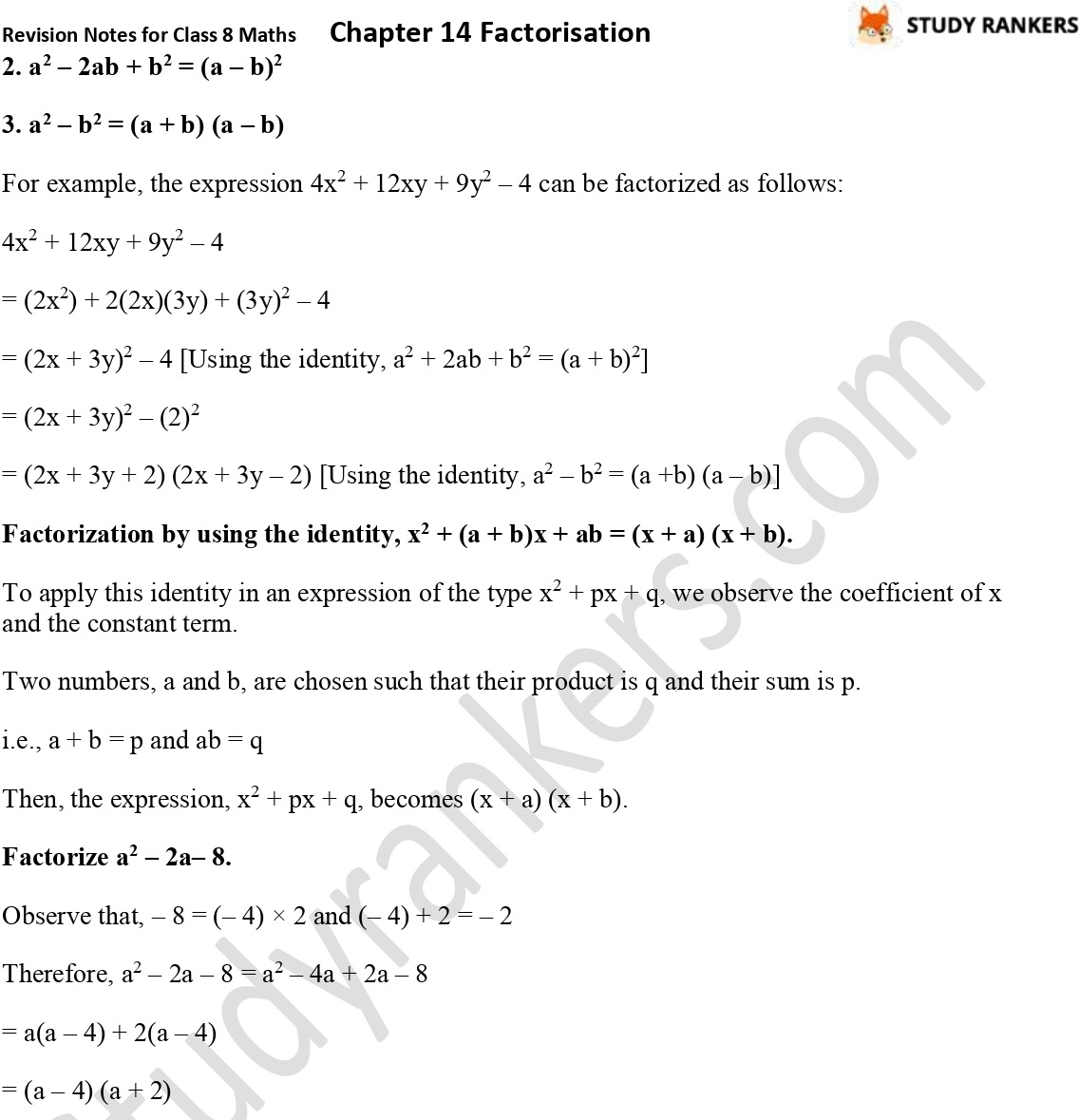## CBSE Revision Notes for Class 8 Chapter 14 Factorisation

Chapter 14 Factorisation Class 8 Revision Notes for Maths can be found here which will be helpful for every Class 8 students in order to understand the chapter more effectively. You should be aware of the concepts given in the chapter that is why Studyrankers has covered all the chapter topicwise in precise manner. You can always look for Class 8 Maths Revision Notes just before examinations which will provide you with the in depth material in no time.

Topics in the chapter:
• Division of any polynomial
• Factorization
• Factorization of expressions by the method of common factors
• Factorize 12p2q + 8pq2 + 18pq
• Factorize 2a2 – b + 2a – ab
• Factorization by using the identity, x2 + (a + b)x + ab = (x + a) (x + b)
• Factorize a2 – 2a– 8.